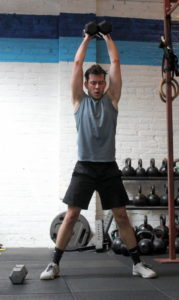## Tuesday, April 23, 2019

### Tuesday, April 23, 2019as many rounds as possible in 12 minutes:
7x box jump, 36/30″ platform
4x bar muscle-up

### 1 Comment

1.–Scores–
Borja G.: 7 rounds + 5x box jump
Jason O: 9 rounds + 2 box jump (sub: pull-up)
Hari P.: 4 rounds + 1 bmu
Charles B.: 5 rounds + 3x box jump, 4 (sub: bar mu, attempts)
Aaron B.: 6 rounds + 1x pull-up (sub: 24″, pull-up)
Al C.: 8 rounds + 5x box jump
Andrew Z.: 6 rounds + 1 box jump (sub: strict pull-up, round 6)
Rami B.: 7 rounds + 2x box jump (sub: pull-up)
Anu B.: 6 rounds + 3x box jump (sub: ctb pull-up)
Morgan W.: 7 rounds (sub: pull-up)
Luke B.: 7 rounds (sub: pull-up)
Jason S.: 9 rounds (sub: 24″, pull-up)
Peter Mc.: 7 rounds + 7x box jump
Chase H.: 6 rounds + 1x bmu
David C.: 6 rounds + 7x box jumps, 5x bmu (sub: 34″, bmu attempts)
Will H.: 6 rounds + 5x body row (sub: 6x true strict body row)
Adrian P.: 5 rounds + 6x box jump (sub: 30″, ctb pull-up)
Walt C.: 5 rounds + 1x strict pull-up, 12 bmu (sub: 34″, bmu attempts/strict pull-up, starting round 4)
Max M.: 8 rounds + 6x pull-up (sub: 2x strict pull-up after round 4)
Dave R.: 9 rounds + 7x box jump
Rebekka E.: 7 rounds + 4x box jump (sub: band)
Alissa G.: 9 rounds + 2x strict pull-up (sub: strict pull-up)
Gabe P.: 7 rounds + 2x pull-up (sub: 2x strict pull-up after round 5)
Will O.: 9 rounds (sub: 2-1 bar mu alternating rounds)
Chenelyn B.: 6 rounds + 3x strict bodyrow (20″; sub: 2x strict bodyrow)
Simon B.: 6 rounds + 2x pull-up (28″; sub: pull-up)
Dave K.: 7 rounds + 7x box jump
Francisco L.: 7 rounds + 7x box jump (sub: 2x strict pull-up after round 6)
Andrew B.: 10 rounds (32″; sub: pull-up)
Charlie S.: 8 rounds + 4x pull-up (sub: ctb pull-up/seated ring pull-up)
Sean Q.: 11 rounds + 5x box jump
Lily: 10 rounds (24″; sub: 7/4x strict bodyrow)
Ralph A.: 6 rounds + 7x box jump (24″; sub: band)
Joya TD.: 9 rounds + 7x box jump (sub: ctb)
Katie G.: 8 rounds + 1x box jump (20″; sub: pull-up)
Zach D.: 6 rounds + 2x box jump (sub: 21x strict sit-up)
Josh B.: 7 rounds + 7x box jump (sub: 3/4x bmu)
Holmes H.: 7 rounds (28″; sub: band)
John S.: 8 rounds + 3x band (30″; sub: band)
Lauren M.: 9 rounds (28″; sub: band)
Joe T.: 9 rounds + 7x box jump
Alli P.: 5 rounds + 1x bmu (sub: 3/4x bmu)
Drew P.: 9 rounds + 5x box jump
Joe R.: 9 rounds + 4x box jump (28″; sub: band)
Gabriel F.: 7 rounds + 7x box jump (32″: sub: strict pull-up)
Sheri F.: 5 rounds + 14x step-up (18″; sub: 2x step-up, 7x strict bodyrow)
John La.: 7 rounds + 7x box jump, 17 (sub: 3x bmu attempt/round)
Julian M.: 8 rounds + 7x box jump (30″; sub: band)
Mel F.: 9 rounds + 7x box jump (sub: 1x bmu/round)
Tara S.: 5 rounds + 7x bmu (sub: 3 bmu attempt/round)
Chris C.: 11 rounds + 2x box jumps (sub: 2 bmu, 2 strict c2b/round)
Ralph B.: 9 rounds + 7x box jump (31″; sub: pull-up)
Seth B.: 8 rounds + 3x box jump (31″; sub: band)
James W.: 6 rounds + 3x pull up (sub: band)
Michail C.: 9 rounds + 2x box jumps (31″; sub: band)
Dan C.: 8 rounds + 5x box jump (31″, sub: c2b)
Brian F.: 6 rounds + 4x box jump (28″, sub: band)
Matt K.: 9 rounds + 2 box jump (30″; sub: pull-up)
Jarod F.: 5 rounds + 7 box jump (sub: pull-up)
Aron S.: 8 rounds + 7 box jump (31″; sub: pull-up)
Patrick J.: 10 rounds + 1 pull-up (31″; sub: pull-up)
Mark Ab.: 9 rounds + 2 box jump (sub: strict pull-up/body row)
Bill G.: 8 rounds + 5 box jumps (32″; sub: c2b)
Kat S.: 9 rounds + 2 pull-up (24″; sub: band)
Krystin H.: 9 rounds + 6 box jumps (24″; sub: band)
Adrienne V.: 9 rounds + 7 box jump (sub: pull-up)
Amy S.: 9 rounds + 1 box jump (sub: 1 bmu/round)
Carl L.: 9 rounds (31″; sub: pull-up)
Lily S.: 7 rounds + 3 pull-up (sub: band)